NEET  >  31 Year NEET Previous Year Questions: Nuclei - 3

# 31 Year NEET Previous Year Questions: Nuclei - 3

Test Description

## 30 Questions MCQ Test Physics Class 12 | 31 Year NEET Previous Year Questions: Nuclei - 3

31 Year NEET Previous Year Questions: Nuclei - 3 for NEET 2023 is part of Physics Class 12 preparation. The 31 Year NEET Previous Year Questions: Nuclei - 3 questions and answers have been prepared according to the NEET exam syllabus.The 31 Year NEET Previous Year Questions: Nuclei - 3 MCQs are made for NEET 2023 Exam. Find important definitions, questions, notes, meanings, examples, exercises, MCQs and online tests for 31 Year NEET Previous Year Questions: Nuclei - 3 below.
Solutions of 31 Year NEET Previous Year Questions: Nuclei - 3 questions in English are available as part of our Physics Class 12 for NEET & 31 Year NEET Previous Year Questions: Nuclei - 3 solutions in Hindi for Physics Class 12 course. Download more important topics, notes, lectures and mock test series for NEET Exam by signing up for free. Attempt 31 Year NEET Previous Year Questions: Nuclei - 3 | 30 questions in 60 minutes | Mock test for NEET preparation | Free important questions MCQ to study Physics Class 12 for NEET Exam | Download free PDF with solutions
 1 Crore+ students have signed up on EduRev. Have you?
31 Year NEET Previous Year Questions: Nuclei - 3 - Question 1

### A radioactive sample with a half life of 1 monthhas the label : ‘Activity = 2 micro curies on 1–8–1991. What would be its activity two monthsearlier ? 

Detailed Solution for 31 Year NEET Previous Year Questions: Nuclei - 3 - Question 1

In two half lives, the activity becomes one fourth.
Activity on 1–8–91 was 2 micro–curie
∴ Activity before two months, 4 × 2 micro-curie = 8 micro curie

31 Year NEET Previous Year Questions: Nuclei - 3 - Question 2

### The nucleus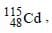after two successive β – decay will give

Detailed Solution for 31 Year NEET Previous Year Questions: Nuclei - 3 - Question 2

Two successive β decay increase the charge number by 2. Therefore, (d) is correct.

31 Year NEET Previous Year Questions: Nuclei - 3 - Question 3

### A radioactive element has half life period 800 years. After 6400 years what amount will remain? 

Detailed Solution for 31 Year NEET Previous Year Questions: Nuclei - 3 - Question 3

No. of half lives,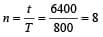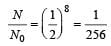31 Year NEET Previous Year Questions: Nuclei - 3 - Question 4

An element A decays into element C by a two step process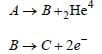then            

Detailed Solution for 31 Year NEET Previous Year Questions: Nuclei - 3 - Question 4

From eqn. (ii), B has 2 units of charge more than C.
From eqn. (i), A loses 2 units of charge by emission of alpha particle. Hence, A and C are isotopes as their charge numbers are same.

31 Year NEET Previous Year Questions: Nuclei - 3 - Question 5

Ultraviolet radiations of 6.2 eV falls on analuminium surface. K.E. of fastest electronemitted is (work function = 4.2 eV) 

Detailed Solution for 31 Year NEET Previous Year Questions: Nuclei - 3 - Question 5

K.E. of fastest electron = E – W0 = 6.2 – 4.2 = 2.0 eV
= 2 × 1.6 × 10–19 = 3.2 × 10– 19 J

31 Year NEET Previous Year Questions: Nuclei - 3 - Question 6

Curie is a unit of      

Detailed Solution for 31 Year NEET Previous Year Questions: Nuclei - 3 - Question 6

Curie is a unit of radioactivity.

31 Year NEET Previous Year Questions: Nuclei - 3 - Question 7

The average binding energy of a nucleon insidean atomic nucleus is about      

Detailed Solution for 31 Year NEET Previous Year Questions: Nuclei - 3 - Question 7

Average B.E./nucleon in nuclei is of the order of 8 MeV.

31 Year NEET Previous Year Questions: Nuclei - 3 - Question 8

The nuclei 6C13 and 7N14 can be described as              

Detailed Solution for 31 Year NEET Previous Year Questions: Nuclei - 3 - Question 8

As 6C13 and 7N14 have same no. of neutrons (13 – 6 =7 for C and 14 – 7 =7 for N), so they are isotones.

31 Year NEET Previous Year Questions: Nuclei - 3 - Question 9

Which of the following statements is true fornuclear forces?  

Detailed Solution for 31 Year NEET Previous Year Questions: Nuclei - 3 - Question 9

Nuclear forces are short range attractive forces which balance the repulsive forces between the protons inside the nucleus.

31 Year NEET Previous Year Questions: Nuclei - 3 - Question 10

The ratio of the radii of the nuclei 13Al27 and 52Te125 is approximately 

Detailed Solution for 31 Year NEET Previous Year Questions: Nuclei - 3 - Question 10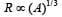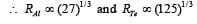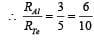31 Year NEET Previous Year Questions: Nuclei - 3 - Question 11

The nucleus 126C absorbs an energetic neutronand emits a beta particle (β). The resultingnucleus is 

Detailed Solution for 31 Year NEET Previous Year Questions: Nuclei - 3 - Question 11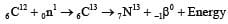31 Year NEET Previous Year Questions: Nuclei - 3 - Question 12

The constituents of atomic nuclei are believedto be  

Detailed Solution for 31 Year NEET Previous Year Questions: Nuclei - 3 - Question 12

Nucleus contains only neutrons and protons.

31 Year NEET Previous Year Questions: Nuclei - 3 - Question 13

The half life of radium is 1600 years. The fractionof a sample of radium that would remain after6400 years     

Detailed Solution for 31 Year NEET Previous Year Questions: Nuclei - 3 - Question 13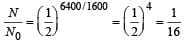31 Year NEET Previous Year Questions: Nuclei - 3 - Question 14

An electron with (rest mass m0) moves with a speed of 0.8 c. Its mass when it moves with this speed is    

Detailed Solution for 31 Year NEET Previous Year Questions: Nuclei - 3 - Question 14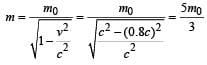31 Year NEET Previous Year Questions: Nuclei - 3 - Question 15

In the nucleus of 11Na23, the number of protons,neutrons and electrons are 

Detailed Solution for 31 Year NEET Previous Year Questions: Nuclei - 3 - Question 15

Z = 11 i.e., number of protons = 11, A = 23
∴ Number of neutrons = A – Z = 12
Number of electron = 0 (No electron in nucleus) Therefore 11, 12, 0.

31 Year NEET Previous Year Questions: Nuclei - 3 - Question 16

If the nuclear force between two protons, two neutrons and between proton and neutron is denoted by Fpp, Fnn and Fpn respectively, then 

Detailed Solution for 31 Year NEET Previous Year Questions: Nuclei - 3 - Question 16

Nuclear force is the same between any two nucleons.

31 Year NEET Previous Year Questions: Nuclei - 3 - Question 17

Solar energy is due to 

Detailed Solution for 31 Year NEET Previous Year Questions: Nuclei - 3 - Question 17

Fusion reaction.

31 Year NEET Previous Year Questions: Nuclei - 3 - Question 18

The energy equivalent of one atomic mass unitis 

Detailed Solution for 31 Year NEET Previous Year Questions: Nuclei - 3 - Question 18

1 a.m.u = 931 MeV

31 Year NEET Previous Year Questions: Nuclei - 3 - Question 19

The mass of α-particle is 

Detailed Solution for 31 Year NEET Previous Year Questions: Nuclei - 3 - Question 19

α-particle = 2He4 . It contains 2p and 2n.
As some mass is converted into B.E., therefore, mass of α particle is slightly less than the sum of the masses of 2p and 2n.

31 Year NEET Previous Year Questions: Nuclei - 3 - Question 20

The mass density of a nucleus varies with massnumber A as 

Detailed Solution for 31 Year NEET Previous Year Questions: Nuclei - 3 - Question 20

The nuclear radius r varies with mass number A according to the relation
r ∝ A1/3 or A ∝ r3

Now,  density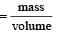Further, mass ∝ A and volume ∝ r3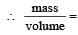constant

31 Year NEET Previous Year Questions: Nuclei - 3 - Question 21

In a given reaction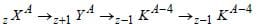Detailed Solution for 31 Year NEET Previous Year Questions: Nuclei - 3 - Question 21

Increase of charge number. by 1 indicates β emission. Decrease of mass number by 4 and charge number by 2 indicates α emission.
No change of mass number and charge number indicates γ-emission.

31 Year NEET Previous Year Questions: Nuclei - 3 - Question 22

The binding energy per nucleon is maximum in case of

Detailed Solution for 31 Year NEET Previous Year Questions: Nuclei - 3 - Question 22

From B.E. curve, the curve reaches peak for
26 Fe56

31 Year NEET Previous Year Questions: Nuclei - 3 - Question 23

Energy released in the fission of a single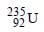nucleus is 200 MeV. The fission rate of afilled reactor operating at a power level of 5 W is            

Detailed Solution for 31 Year NEET Previous Year Questions: Nuclei - 3 - Question 23

Fission rate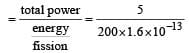= 1.56 × 1011 s–1

31 Year NEET Previous Year Questions: Nuclei - 3 - Question 24

If the binding energy per nucleon in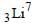and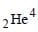nuclei are respectively 5.60 MeV and 7.06 MeV, then the energy of proton in the reaction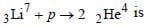Detailed Solution for 31 Year NEET Previous Year Questions: Nuclei - 3 - Question 24

Applying principle of energy conservation, Energy of proton = total B.E. of 2α– energy of Li7
= 8 × 7.06 – 7 × 5.6
= 56.48 – 39.2 = 17.28 MeV

31 Year NEET Previous Year Questions: Nuclei - 3 - Question 25

Heavy water is used as a moderator in a nuclearreactor. The function of the moderator is

Detailed Solution for 31 Year NEET Previous Year Questions: Nuclei - 3 - Question 25

Moderator slows down the neutrons to thermal energies.

31 Year NEET Previous Year Questions: Nuclei - 3 - Question 26

The mass number of He is 4 and that for sulphur is 32. The radius of sulphur nuclei is larger than that of helium by [1994, 1995]

Detailed Solution for 31 Year NEET Previous Year Questions: Nuclei - 3 - Question 26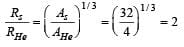31 Year NEET Previous Year Questions: Nuclei - 3 - Question 27

The nature of ions knocked out from hotsurfaces is 

Detailed Solution for 31 Year NEET Previous Year Questions: Nuclei - 3 - Question 27

Ion is an atom or a group of atoms that has lost or gained electrons having negative or positive charge respectively. Thus, the ions knocked out from hot surfaces is electrons.

31 Year NEET Previous Year Questions: Nuclei - 3 - Question 28

What is the respective number of α and β particles emitted in the following radioactive decay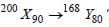?    

Detailed Solution for 31 Year NEET Previous Year Questions: Nuclei - 3 - Question 28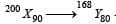We know that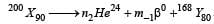Therefore, in this process,

200 = 4n + 168 or  n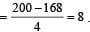Also, 90 = 2n – m + 80
or,  m = 2n + 80 – 90 = (2 × 8 + 80 – 90) = 6.
Thus,  respective number of α and β-particles will be 8 and 6.

31 Year NEET Previous Year Questions: Nuclei - 3 - Question 29

The count rate of a Geiger Muller counter for theradiation of a radioactive material of half-life 30minutes decreases to 5 sec–1 after 2 hours. Theinitial count rate was 

Detailed Solution for 31 Year NEET Previous Year Questions: Nuclei - 3 - Question 29

Half-life = 30 minutes; Rate of decrease (N) = 5 per second and total time = 2 hours = 120 minutes. Relation for initial and final count rate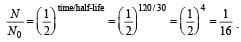Therefore, N0 = 16 × N = 16 × 5 = 80 s–1.

31 Year NEET Previous Year Questions: Nuclei - 3 - Question 30

A nucleus ruptures into two nuclear parts, whichhave their velocity ratio equal to 2:1. What willbe the ratio of their nuclear size (nuclear radius)?        

Detailed Solution for 31 Year NEET Previous Year Questions: Nuclei - 3 - Question 30

Applying law of conservation of momentum,
m1v1 = m2v2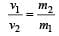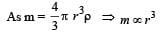Hence,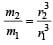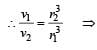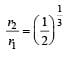## Physics Class 12

157 videos|452 docs|213 tests
 Use Code STAYHOME200 and get INR 200 additional OFF Use Coupon Code
Information about 31 Year NEET Previous Year Questions: Nuclei - 3 Page
In this test you can find the Exam questions for 31 Year NEET Previous Year Questions: Nuclei - 3 solved & explained in the simplest way possible. Besides giving Questions and answers for 31 Year NEET Previous Year Questions: Nuclei - 3, EduRev gives you an ample number of Online tests for practice

## Physics Class 12

157 videos|452 docs|213 tests

### How to Prepare for NEET

Read our guide to prepare for NEET which is created by Toppers & the best Teachers